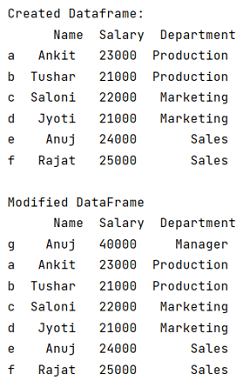# How to insert rows in pandas DataFrame?

Given a Pandas DataFrame, we have to insert rows in it. By Pranit Sharma Last updated : September 22, 2023

Rows in pandas are the different cell (column) values which are aligned horizontally and also provides uniformity. Each row can have same or different value. Rows are generally marked with the index number but in pandas we can also assign index name according to the needs. In pandas, we can create, read, update and delete a column or row value.

## Inserting rows in pandas DataFrame

To insert rows in pandas DataFrame - We will first create a DataFrame and then we will concat it with the previous DataFrame using the pandas.concat() method, this method is used to concatenate pandas objects such as DataFrames and Series.

Note

To work with pandas, we need to import pandas package first, below is the syntax:

```import pandas as pd
```

Let us understand with the help of an example,

## Python program to insert rows in pandas DataFrame

```# Import pandas Package
import pandas as pd

# Creating dictionary
d = {
'Name':['Ankit', 'Tushar', 'Saloni','Jyoti', 'Anuj', 'Rajat'],
'Salary':[23000, 21000, 22000, 21000, 24000, 25000],
'Department':['Production', 'Production', 'Marketing', 'Marketing', 'Sales', 'Sales']
}

# Creating a Dataframe
df = pd.DataFrame(d,index = ['a', 'b', 'c', 'd', 'e', 'f'])

print("Created Dataframe:\n", df,"\n")

# Creating a new Dataframe with one row
new_df = pd.DataFrame({
'Name':['Anuj'],
'Salary':,
'Department':['Manager']},index=['g'])

# Concatenating new dataframe and old dataframe
result = pd.concat([new_df,df])

# Display result
print("Modified DataFrame\n",result)
```

### Output

The output of the above program is: